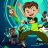# Safe Hull Moving Average Indicator#### BenTen

Staff
VIP
Warehouse

> This indicator is the standard colored Hull, expect that it has a neutral color. When the Hull does not match the MACD, it colors it yellow. We assume that when the Hull color is Green and Red, those are the safe periods.thinkScript Code
Rich (BB code):
``````input price = close;
input length = 100;
input displace = 0;

input FastLength = 180;
input SlowLength = 208;
input MACDLength = 72;

input AverageType = {SMA, default EMA};

def macd = MACD(FastLength, SlowLength, MACDLength, AverageType).Diff;

def halflength = Ceil(length / 2);
def sqrtlength = Ceil(Sqrt(length));
def val = 2 * wma(price, halflength) - wma(price, length);

plot HMA = wma(val, sqrtlength)[-displace];
HMA.SetDefaultColor(GetColor(1));

HMA.SetLineWeight(2);
HMA.DefineColor("UP", Color.BLUE);
HMA.DefineColor("DOWN", Color.RED);
HMA.DefineColor("NEUTRAL", Color.ORANGE);

def isUP;
def isDOWN;
def isNEUTRAL;

if HMA > HMA and macd >= 0 then {
isUP = yes;
isDOWN = no;
isNEUTRAL = no;
} else {
if HMA < HMA and macd <= 0 then {
isUP = no;
isDOWN = yes;
isNEUTRAL = no;
} else {
isUP = no;
isDOWN = no;
isNEUTRAL = yes;
}
}

HMA.AssignValueColor(if isUP then HMA.color("UP") else if isDOWN then HMA.color("DOWN") else HMA.color("NEUTRAL"));``````
Shareable Script
http://tos.mx/nbwaBU

Last edited:
•Indicators 23